# Tensorflow Python API 翻译（math_ops）（第二部分）

## 该章介绍有关数学符号操作的API

###### 减少元素操作

TensorFlow提供了一些操作，你可以用它来执行常见的数学运算，以此减少张量的维度。

`tf.reduce_sum(input_tensor, reduction_indices=None, keep_dims=False, name=None)`

``````# 'x' is [[1, 1, 1]]
#         [1, 1, 1]]
tf.reduce_sum(x) ==> 6
tf.reduce_sum(x, 0) ==> [2, 2, 2]
tf.reduce_sum(x, 1) ==> [3, 3]
tf.reduce_sum(x, 1, keep_dims=True) ==> [, ]
tf.reduce_sum(x, [0, 1]) ==> 6复制代码``````

``````#!/usr/bin/env python
# -*- coding: utf-8 -*-

import tensorflow as tf
import numpy as np

a = tf.constant(np.random.rand(3,4))
c = tf.reduce_sum(a, 1, keep_dims = True)
sess = tf.Session()
print sess.run(c)
sess.close()复制代码``````

* `input_tensor`: 一个累加的`Tensor`，它应该是数字类型。
* `reduction_indices`: 指定累加的维度。如果是`None`，那么累加所有的元素。
* `keep_dims`: 如果是`True`，那么指定维度中的元素累加返回一个秩为1的`Tensor`。如果是`False`，那么返回一个累加的标量。
* `name`:（可选）为这个操作取一个名字。

* 一个累加的`Tensor`

`tf.reduce_prod(input_tensor, reduction_indices=None, keep_dims=False, name=None)`

``````#!/usr/bin/env python
# -*- coding: utf-8 -*-

import tensorflow as tf
import numpy as np

a = tf.constant([[2,3,1],[4,5,1]])
c = tf.reduce_prod(a, 0)
sess = tf.Session()
print sess.run(c)
sess.close()复制代码``````

* `input_tensor`: 一个累乘的`Tensor`，它应该是数字类型。
* `reduction_indices`: 指定累乘的维度。如果是`None`，那么累乘所有的元素。
* `keep_dims`: 如果是`True`，那么指定维度中的元素累乘返回一个秩为1的`Tensor`。如果是`False`，那么返回一个累乘的标量。
* `name`:（可选）为这个操作取一个名字。

* 一个累乘的`Tensor`

`tf.reduce_min(input_tensor, reduction_indices=None, keep_dims=False, name=None)`

``````#!/usr/bin/env python
# -*- coding: utf-8 -*-

import tensorflow as tf
import numpy as np

a = tf.constant([[2,3,2],[4,5,1]])
c = tf.reduce_min(a, 0)
sess = tf.Session()
print sess.run(c)
sess.close()复制代码``````

* `input_tensor`: 一个需要处理的`Tensor`，它应该是数字类型。
* `reduction_indices`: 指定需要查找最小值的维度。如果是`None`，那么从所有的元素中找最小值。
* `keep_dims`: 如果是`True`，那么指定维度中的最小值返回一个秩为1的`Tensor`。如果是`False`，那么返回一个最小值的标量。
* `name`:（可选）为这个操作取一个名字。

* 一个处理之后的`Tensor`

`tf.reduce_max(input_tensor, reduction_indices=None, keep_dims=False, name=None)`

``````#!/usr/bin/env python
# -*- coding: utf-8 -*-

import tensorflow as tf
import numpy as np

a = tf.constant([[2,3,2],[4,5,1]])
c = tf.reduce_max(a, 0)
sess = tf.Session()
print sess.run(c)
sess.close()复制代码``````

* `input_tensor`: 一个需要处理的`Tensor`，它应该是数字类型。
* `reduction_indices`: 指定需要查找最大值的维度。如果是`None`，那么从所有的元素中找最大值。
* `keep_dims`: 如果是`True`，那么指定维度中的最大值返回一个秩为1的`Tensor`。如果是`False`，那么返回一个最大值的标量。
* `name`:（可选）为这个操作取一个名字。

* 一个处理之后的`Tensor`

`tf.reduce_mean(input_tensor, reduction_indices=None, keep_dims=False, name=None)`

``````# 'x' is [[1., 1. ]]
#         [2., 2.]]
tf.reduce_mean(x) ==> 1.5
tf.reduce_mean(x, 0) ==> [1.5, 1.5]
tf.reduce_mean(x, 1) ==> [1.,  2.]复制代码``````

``````#!/usr/bin/env python
# -*- coding: utf-8 -*-

import tensorflow as tf
import numpy as np

a = tf.constant([[2,3,2],[4,5,1]], tf.float32)
c = tf.reduce_mean(a, 0)
sess = tf.Session()
print sess.run(c)
sess.close()复制代码``````

* `input_tensor`: 一个需要处理的`Tensor`，它应该是数字类型。
* `reduction_indices`: 指定需要查找平均值的维度。如果是`None`，那么从所有的元素中找平均值。
* `keep_dims`: 如果是`True`，那么指定维度中的平均值返回一个秩为1的`Tensor`。如果是`False`，那么返回一个平均值的标量。
* `name`:（可选）为这个操作取一个名字。

* 一个处理之后的`Tensor`

`tf.reduce_all(input_tensor, reduction_indices=None, keep_dims=False, name=None)`

``````# 'x' is [[True,  True]]
#         [False, False]]
tf.reduce_all(x) ==> False
tf.reduce_all(x, 0) ==> [False, False]
tf.reduce_all(x, 1) ==> [True, False]

``````#!/usr/bin/env python
# -*- coding: utf-8 -*-

import tensorflow as tf
import numpy as np

a = tf.constant([[False, False,True],[False,True,True]])
c = tf.reduce_all(a, 0)
sess = tf.Session()
print sess.run(c)
sess.close()复制代码``````

* `input_tensor`: 一个需要处理的`Tensor`，它应该是数字类型。
* `reduction_indices`: 指定需要查找逻辑与值的维度。如果是`None`，那么从所有的元素中找逻辑与值。
* `keep_dims`: 如果是`True`，那么指定维度中的逻辑与值返回一个秩为1的`Tensor`。如果是`False`，那么返回一个逻辑与值的标量。
* `name`:（可选）为这个操作取一个名字。

* 一个处理之后的`Tensor`

`tf.reduce_any(input_tensor, reduction_indices=None, keep_dims=False, name=None)`

``````# 'x' is [[True,  True]]
#         [False, False]]
tf.reduce_all(x) ==> False
tf.reduce_all(x, 0) ==> [True, True]
tf.reduce_all(x, 1) ==> [True, False]

``````#!/usr/bin/env python
# -*- coding: utf-8 -*-

import tensorflow as tf
import numpy as np

a = tf.constant([[False, False,True],[False,True,True]])
c = tf.reduce_any(a, 0)
sess = tf.Session()
print sess.run(c)
sess.close()复制代码``````

* `input_tensor`: 一个需要处理的`Tensor`，它应该是数字类型。
* `reduction_indices`: 指定需要查找逻辑或值的维度。如果是`None`，那么从所有的元素中找逻辑或值。
* `keep_dims`: 如果是`True`，那么指定维度中的逻辑或值返回一个秩为1的`Tensor`。如果是`False`，那么返回一个逻辑或值的标量。
* `name`:（可选）为这个操作取一个名字。

* 一个处理之后的`Tensor`

`tf.accumulate_n(inputs, shape=None, tensor_dtype=None, name=None)`

``````# tensor 'a' is [[1, 2], [3, 4]
# tensor `b` is [[5, 0], [0, 6]]
tf.accumulate_n([a, b, a]) ==> [[7, 4], [6, 14]]

# Explicitly pass shape and type
tf.accumulate_n([a, b, a], shape=[2, 2], tensor_dtype=tf.int32)
==> [[7, 4], [6, 14]]复制代码``````

``````#!/usr/bin/env python
# -*- coding: utf-8 -*-

import tensorflow as tf
import numpy as np

a = tf.constant([[1, 2], [3, 4]])
b = tf.constant([[5, 0], [0, 6]])
c = tf.accumulate_n([a,b,a], shape = [2,2])
sess = tf.Session()
print sess.run(c)
sess.close()复制代码``````

* `inputs`: 一个需要处理的`Tensor`列表，其中每一个`tensor`都必须拥有相同的数据维度和数据类型。
* `shape`: `inputs`的数据维度。
* `tensor_dtype`: `inputs`的数据类型。
* `name`:（可选）为这个操作取一个名字。

* 一个`Tensor`，数据维度和数据类型都和`inputs`相同。

* 如果`inputs`中每一个`tensor`的数据维度不一样，或者推测的数据维度或数据类型不正确，那么都会抛出异常。

###### 分割操作

TensorFlow提供了一些操作，你可以使用基本的算术运算来分割输入的`tensor`。这里的分割操作是沿着第一个维度的一个分区，等价于这里定义了一个从第一个维度到第`segment_ids`维度的一个映射。`segment_ids`张量的长度必须和需要分割的`tensor`的第一维度的尺寸`d0`一样，其中`segment_ids`中的编号从`0``k`，并且`k < d0`。举个例子，如果我们需要分割的`tensor`是一个矩阵，那么`segment_ids`的映射就指向矩阵的每一行。

``````c = tf.constant([[1,2,3,4], [-1,-2,-3,-4], [5,6,7,8]])
tf.segment_sum(c, tf.constant([0, 0, 1]))
==>  [[0 0 0 0]
[5 6 7 8]]复制代码``````

`tf.segment_sum(data, segment_ids, name=None)`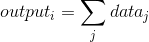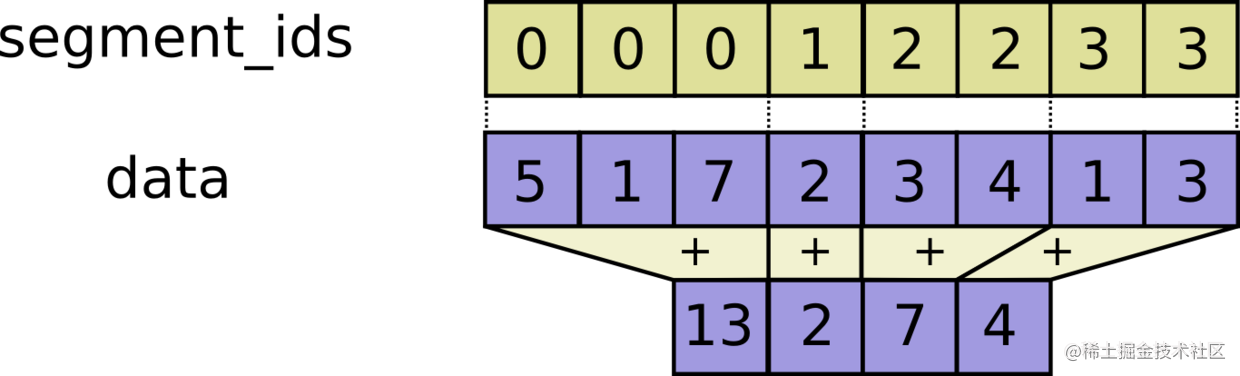``````#!/usr/bin/env python
# -*- coding: utf-8 -*-

import tensorflow as tf
import numpy as np

a = tf.constant([[1,2,3,4], [-1,-2,-3,-4], [5,6,7,8], [-1,-2,-3,-4]])
c = tf.segment_sum(a, tf.constant([0, 0, 1, 2]))
sess = tf.Session()
print sess.run(c)
sess.close()复制代码``````

* `data`: 一个`Tensor`，数据类型必须是以下之一：`float32``float64``int32``int64``uint8``int16``int8`
* `segment_ids`: 一个`tensor`，数据类型必须是`int32`或者`int64`，数据维度是一维的，并且长度和`data`第一维度的长度相同。里面的值是从`0``k`的有序排列，但是可以重复。
* `name`:（可选）为这个操作取一个名字。

* 一个`Tensor`，数据类型和`data`相同，数据的第一维度是`k`，其余维度和`data`相同。

`tf.segment_prod(data, segment_ids, name=None)`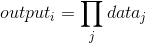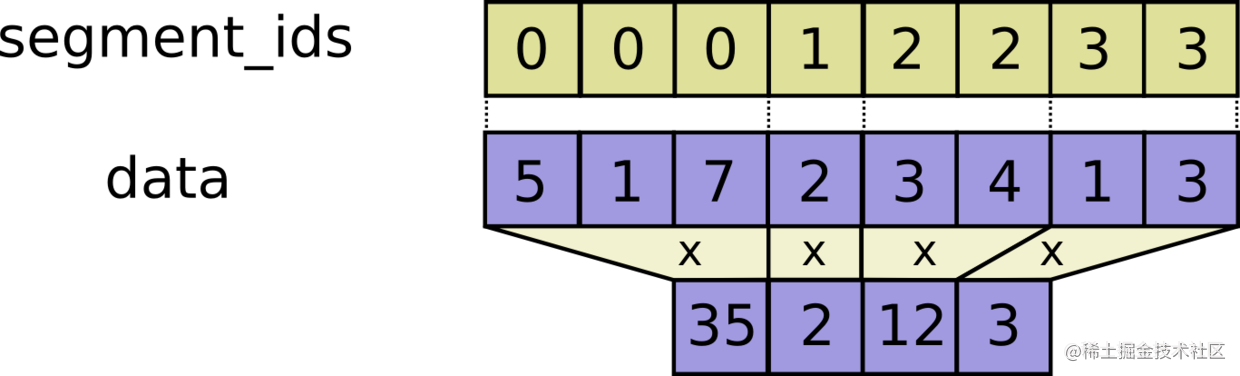``````#!/usr/bin/env python
# -*- coding: utf-8 -*-

import tensorflow as tf
import numpy as np

a = tf.constant([[1,2,3,4], [-1,-2,-3,-4], [5,6,7,8], [-1,-2,-3,-4]])
c = tf.segment_prod(a, tf.constant([0, 0, 1, 2]))
sess = tf.Session()
print sess.run(c)
sess.close()复制代码``````

* `data`: 一个`Tensor`，数据类型必须是以下之一：`float32``float64``int32``int64``uint8``int16``int8`
* `segment_ids`: 一个`tensor`，数据类型必须是`int32`或者`int64`，数据维度是一维的，并且长度和`data`第一维度的长度相同。里面的值是从`0``k`的有序排列，但是可以重复。
* `name`:（可选）为这个操作取一个名字。

* 一个`Tensor`，数据类型和`data`相同，数据的第一维度是`k`，其余维度和`data`相同。

`tf.segment_min(data, segment_ids, name=None)`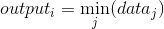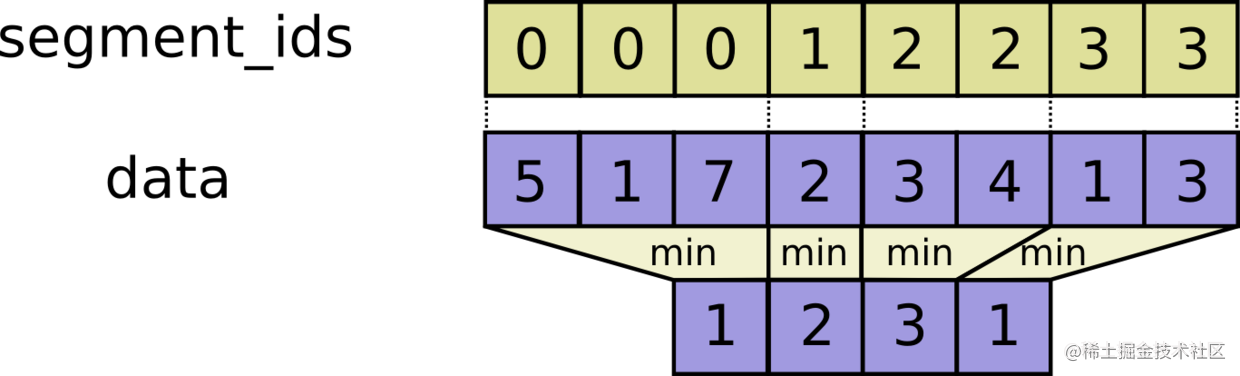``````#!/usr/bin/env python
# -*- coding: utf-8 -*-

import tensorflow as tf
import numpy as np

a = tf.constant([[1,2,3,4], [-1,-2,-3,-4], [5,6,7,8], [-1,-2,-3,-4]])
c = tf.segment_min(a, tf.constant([0, 0, 1, 2]))
sess = tf.Session()
print sess.run(c)
sess.close()复制代码``````

* `data`: 一个`Tensor`，数据类型必须是以下之一：`float32``float64``int32``int64``uint8``int16``int8`
* `segment_ids`: 一个`tensor`，数据类型必须是`int32`或者`int64`，数据维度是一维的，并且长度和`data`第一维度的长度相同。里面的值是从`0``k`的有序排列，但是可以重复。
* `name`:（可选）为这个操作取一个名字。

* 一个`Tensor`，数据类型和`data`相同，数据的第一维度是`k`，其余维度和`data`相同。

`tf.segment_max(data, segment_ids, name=None)`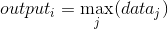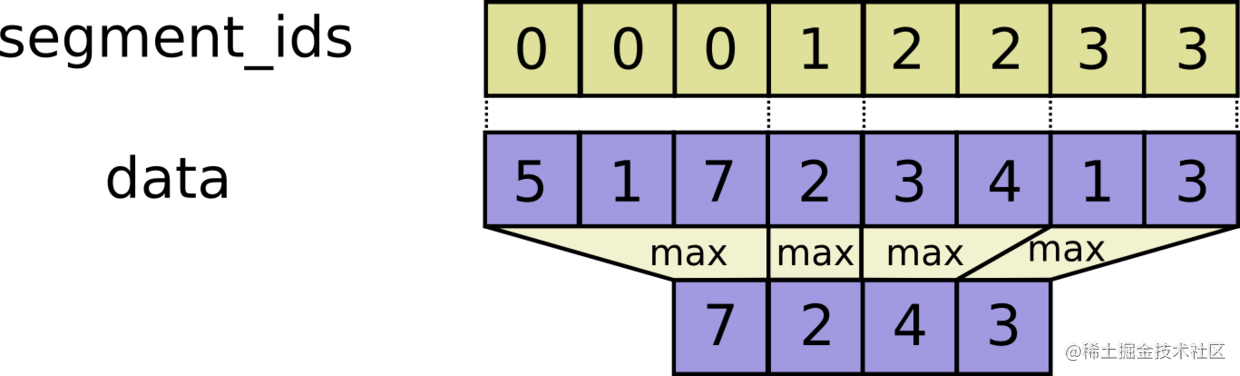``````#!/usr/bin/env python
# -*- coding: utf-8 -*-

import tensorflow as tf
import numpy as np

a = tf.constant([[1,2,3,4], [-1,-2,-3,-4], [5,6,7,8], [-1,-2,-3,-4]])
c = tf.segment_max(a, tf.constant([0, 0, 1, 2]))
sess = tf.Session()
print sess.run(c)
sess.close()复制代码``````

* `data`: 一个`Tensor`，数据类型必须是以下之一：`float32``float64``int32``int64``uint8``int16``int8`
* `segment_ids`: 一个`tensor`，数据类型必须是`int32`或者`int64`，数据维度是一维的，并且长度和`data`第一维度的长度相同。里面的值是从`0``k`的有序排列，但是可以重复。
* `name`:（可选）为这个操作取一个名字。

* 一个`Tensor`，数据类型和`data`相同，数据的第一维度是`k`，其余维度和`data`相同。

`tf.segment_mean(data, segment_ids, name=None)`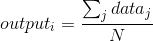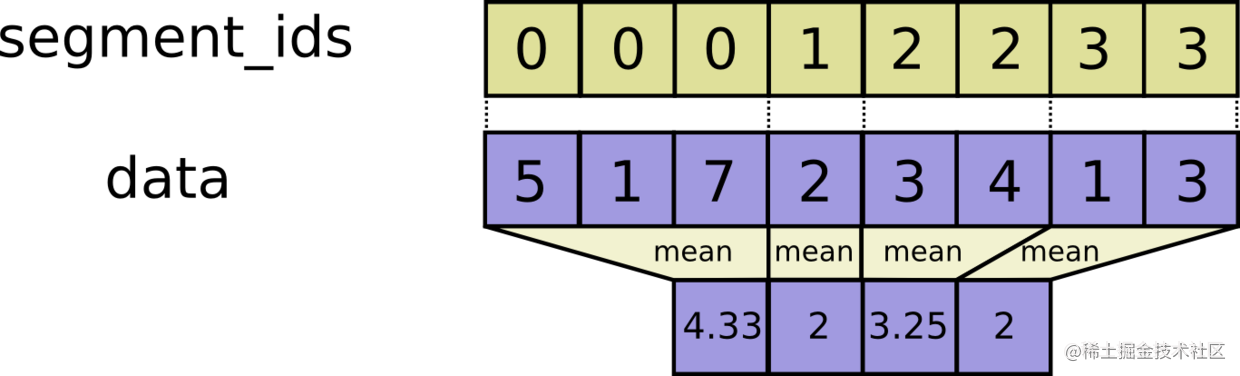``````#!/usr/bin/env python
# -*- coding: utf-8 -*-

import tensorflow as tf
import numpy as np

a = tf.constant([[1,2,3,4], [-1,-2,-3,-4], [5,6,7,8], [-1,-2,-3,-4]])
c = tf.segment_mean(a, tf.constant([0, 0, 1, 2]))
sess = tf.Session()
print sess.run(c)
sess.close()复制代码``````

* `data`: 一个`Tensor`，数据类型必须是以下之一：`float32``float64``int32``int64``uint8``int16``int8`
* `segment_ids`: 一个`tensor`，数据类型必须是`int32`或者`int64`，数据维度是一维的，并且长度和`data`第一维度的长度相同。里面的值是从`0``k`的有序排列，但是可以重复。
* `name`:（可选）为这个操作取一个名字。

* 一个`Tensor`，数据类型和`data`相同，数据的第一维度是`k`，其余维度和`data`相同。

`tf.unsorted_segment_sum(data, segment_ids, num_segments, name=None)`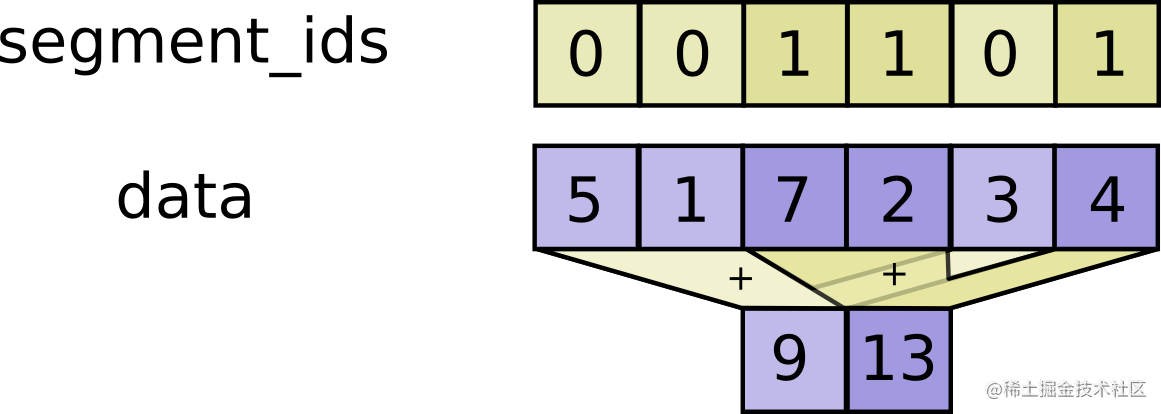``````#!/usr/bin/env python
# -*- coding: utf-8 -*-

import tensorflow as tf
import numpy as np

a = tf.constant([[1,2,3,4], [-1,-2,-3,-4], [5,6,7,8], [-1,-2,-3,-4]])
c = tf.unsorted_segment_sum(a, tf.constant([0, 0, 1, 1]), 2)
sess = tf.Session()
print sess.run(c)
sess.close()复制代码``````

* `data`: 一个`Tensor`，数据类型必须是以下之一：`float32``float64``int32``int64``uint8``int16``int8`
* `segment_ids`: 一个`tensor`，数据类型必须是`int32`或者`int64`，数据维度是一维的，并且长度和`data`第一维度的长度相同。
* `num_segments`: 一个`tensor`，数据类型是`int32`
* `name`:（可选）为这个操作取一个名字。

* 一个`Tensor`，数据类型和`data`相同，数据的第一维度是`num_segments`，其余维度和`data`相同。

`tf.sparse_segment_sum(data, indices, segment_ids, name=None)`

``````c = tf.constant([[1,2,3,4], [-1,-2,-3,-4], [5,6,7,8]])

# Select two rows, one segment.
tf.sparse_segment_sum(c, tf.constant([0, 1]), tf.constant([0, 0]))
==> [[0 0 0 0]]

# Select two rows, two segment.
tf.sparse_segment_sum(c, tf.constant([0, 1]), tf.constant([0, 1]))
==> [[ 1  2  3  4]
[-1 -2 -3 -4]]

# Select all rows, two segments.
tf.sparse_segment_sum(c, tf.constant([0, 1, 2]), tf.constant([0, 0, 1]))
==> [[0 0 0 0]
[5 6 7 8]]

# Which is equivalent to:
tf.segment_sum(c, tf.constant([0, 0, 1]))复制代码``````

``````#!/usr/bin/env python
# -*- coding: utf-8 -*-

import tensorflow as tf
import numpy as np

a = tf.constant([[1,2,3,4], [-1,-2,-3,-4], [5,6,7,8], [-1,-2,-3,-4]])
c = tf.sparse_segment_sum(a, tf.constant([0, 1, 1, 2]), tf.constant([0, 0, 1, 2]))
sess = tf.Session()
print sess.run(c)
sess.close()复制代码``````

* `data`: 一个`Tensor`，数据类型必须是以下之一：`float32``float64``int32``int64``uint8``int16``int8`
* `indices`: 一个`tensor`，数据类型是`int32`，数据维度是一维的，长度和`segment_ids`相同。
* `segment_ids`: 一个`tensor`，数据类型必须是`int32`，数据维度是一维的。里面的值是有序排列的，但是可以重复。

• `name`:（可选）为这个操作取一个名字。

* 一个`Tensor`，数据类型和`data`相同，数据的第一维度是`k`，其余维度和`data`相同。

`tf.sparse_segment_mean(data, indices, segment_ids, name=None)`

``````#!/usr/bin/env python
# -*- coding: utf-8 -*-

import tensorflow as tf
import numpy as np

a = tf.constant([[1,2,3,4], [-1,-2,-3,-4], [5,6,7,8], [-1,-2,-3,-4]], tf.float32)
c = tf.sparse_segment_mean(a, tf.constant([0, 1, 1, 2]), tf.constant([0, 0, 1, 2]))
sess = tf.Session()
print sess.run(c)
sess.close()复制代码``````

* `data`: 一个`Tensor`，数据类型必须是以下之一：`float32``float64`
* `indices`: 一个`tensor`，数据类型是`int32`，数据维度是一维的，长度和`segment_ids`相同。
* `segment_ids`: 一个`tensor`，数据类型必须是`int32`，数据维度是一维的。里面的值是有序排列的，但是可以重复。

• `name`:（可选）为这个操作取一个名字。

* 一个`Tensor`，数据类型和`data`相同，数据的第一维度是`k`，其余维度和`data`相同。

###### 序列比较和索引函数

TensorFlow提供了一些操作，你可以使用这些函数去处理序列比较和索引提取，并且添加到你的图中。你可以使用这些函数去确定一些序列之间的差异，以及确定`tensor`中一些特定的值的索引。

`tf.argmin(input, dimension, name=None)`

``````#!/usr/bin/env python
# -*- coding: utf-8 -*-

import tensorflow as tf
import numpy as np

a = tf.constant([[11,22,3,4], [2,6,3,1]])
c = tf.argmin(a, 1)
sess = tf.Session()
print sess.run(c)
sess.close()复制代码``````

* `input`: 一个`Tensor`，数据类型必须是以下之一：`float32``float64``int64``int32``uint8``uint16``int8``complex64``qint8``qint32`
* `dimension`: 一个`tensor`，数据类型是`int32``0 <= dimension < rank(input)`。这个参数选定了需要合并处理的哪个维度。如果输入`input`是一个向量，那么我们取`dimension = 0`
* `name`:（可选）为这个操作取一个名字。

* 一个`Tensor`，数据类型是`int64`

`tf.argmax(input, dimension, name=None)`

``````#!/usr/bin/env python
# -*- coding: utf-8 -*-

import tensorflow as tf
import numpy as np

a = tf.constant([[11,22,3,4], [2,6,3,1]])
c = tf.argmax(a, 1)
sess = tf.Session()
print sess.run(c)
sess.close()复制代码``````

* `input`: 一个`Tensor`，数据类型必须是以下之一：`float32``float64``int64``int32``uint8``uint16``int8``complex64``qint8``qint32`
* `dimension`: 一个`tensor`，数据类型是`int32``0 <= dimension < rank(input)`。这个参数选定了需要合并处理的哪个维度。如果输入`input`是一个向量，那么我们取`dimension = 0`
* `name`:（可选）为这个操作取一个名字。

* 一个`Tensor`，数据类型是`int64`

`tf.listdiff(x, y, name=None)`

``out[i] = x[idx[i]] for i in [0, 1, ..., len(out) - 1]复制代码``

``````x = [1, 2, 3, 4, 5, 6]
y = [1, 3, 5]复制代码``````

``````out ==> [2, 4, 6]
idx ==> [1, 3, 5]复制代码``````

``````#!/usr/bin/env python
# -*- coding: utf-8 -*-

import tensorflow as tf
import numpy as np

a = tf.constant([2,6,3,1])
b = tf.constant([11,22,3,4, 8])
c = tf.listdiff(a, b)
sess = tf.Session()
print sess.run(c)
sess.close()复制代码``````

* `x`: 一个一维的`Tensor`，里面的值是需要保留的。
* `y`: 一个一维的`tensor`，数据类型和`x`相同，里面的值是需要去除的。
* `name`:（可选）为这个操作取一个名字。

• `out`: 一个`tensor`，数据类型和`x`相同，数据维度是一维的，里面的元素存在于`x`中，但不存在与`y`中。
• `idx`: 一个`tensor`，数据类型是`int32`，数据维度是一维的，里面的元素表示`out`中的值在原来`x`中的索引位置。

`tf.where(input, name=None)`

``````# 'input' tensor is [[True, False]
#                    [True, False]]
# 'input' has two true values, so output has two coordinates.
# 'input' has rank of 2, so coordinates have two indices.
where(input) ==> [[0, 0],
[1, 0]]

# `input` tensor is [[[True, False]
#                     [True, False]]
#                    [[False, True]
#                     [False, True]]
#                    [[False, False]
#                     [False, True]]]
# 'input' has 5 true values, so output has 5 coordinates.
# 'input' has rank of 3, so coordinates have three indices.
where(input) ==> [[0, 0, 0],
[0, 1, 0],
[1, 0, 1],
[1, 1, 1],
[2, 1, 1]]复制代码``````

``````#!/usr/bin/env python
# -*- coding: utf-8 -*-

import tensorflow as tf
import numpy as np

a = tf.constant([[True, False],[False, True]])
c = tf.where(a)
sess = tf.Session()
print sess.run(c)
sess.close()复制代码``````

* `input`: 一个`Tensor`，数据类型是布尔类型`bool`
* `name`:（可选）为这个操作取一个名字。

• 一个`tensor`，数据类型是`int64`

`tf.unique(x, name=None)`

``y[idx[i]] = x[i] for i in [0, 1,...,rank(x) - 1]复制代码``

``````# tensor 'x' is [1, 1, 2, 4, 4, 4, 7, 8, 8]
y, idx = unique(x)
y ==> [1, 2, 4, 7, 8]
idx ==> [0, 0, 1, 2, 2, 2, 3, 4, 4]复制代码``````

``````#!/usr/bin/env python
# -*- coding: utf-8 -*-

import tensorflow as tf
import numpy as np

a = tf.constant([1, 1, 24, 4, 4, 4, 7, 8, 8])
c, d = tf.unique(a)
sess = tf.Session()
print sess.run(c)
print sess.run(d)
sess.close()复制代码``````

* `x`: 一个`Tensor`，数据维度是一维的。
* `name`:（可选）为这个操作取一个名字。

• `y`: 一个`tensor`，数据类型和`x`相同，数据维度是一维的。
• `idx`: 一个`tensor`，数据类型是`int32`，数据维度是一维的。

`tf.edit_distance(hypothesis, truth, normalize=True, name='edit_distance')`

``````# 'hypothesis' is a tensor of shape `[2, 1]` with variable-length values:
#   (0,0) = ["a"]
#   (1,0) = ["b"]
hypothesis = tf.SparseTensor(
[[0, 0, 0],
[1, 0, 0]],
["a", "b"]
(2, 1, 1))

# 'truth' is a tensor of shape `[2, 2]` with variable-length values:
#   (0,0) = []
#   (0,1) = ["a"]
#   (1,0) = ["b", "c"]
#   (1,1) = ["a"]
truth = tf.SparseTensor(
[[0, 1, 0],
[1, 0, 0],
[1, 0, 1],
[1, 1, 0]]
["a", "b", "c", "a"],
(2, 2, 2))

normalize = True复制代码``````

``````# 'output' is a tensor of shape `[2, 2]` with edit distances normalized
# by 'truth' lengths.
output ==> [[inf, 1.0],  # (0,0): no truth, (0,1): no hypothesis
[0.5, 1.0]]  # (1,0): addition, (1,1): no hypothesis复制代码``````

``````#!/usr/bin/env python
# -*- coding: utf-8 -*-

import tensorflow as tf
import numpy as np

hypothesis = tf.SparseTensor(
[[0, 0, 0],
[1, 0, 0]],
["a", "b"],
(2, 1, 1))

truth = tf.SparseTensor(
[[0, 1, 0],
[1, 0, 0],
[1, 0, 1],
[1, 1, 0]],
["a", "b", "c", "a"],
(2, 2, 2))

c = tf.edit_distance(hypothesis, truth)
sess = tf.Session()
print sess.run(c)
sess.close()复制代码``````

• `hypothesis`: 一个`SparseTensor`，表示猜测的数据序列。
• `truth`: 一个`SparseTensor`，表示真实的数据序列。
• `normalize`: 一个布尔类型，如果设置为`true`，那么最后结果将根据`truth`的长度进行归一化。
• `name`:（可选）为这个操作取一个名字。

• 一个密集`tensor`，其秩为`R-1`。其中，`R`是输入`hypothesis``truth`的秩。

• `类型异常`: 如果`hypothesis``truth`不是`SparseTensor`类型的，那么就会抛出这个异常。

`tf.invert_permutation(x, name=None)`

``y[x[i]] = i for i in [0, 1, ..., len(x) - 1]复制代码``

``````# tensor `x` is [3, 4, 0, 2, 1]
invert_permutation(x) ==> [2, 4, 3, 0, 1]复制代码``````

``````#!/usr/bin/env python
# -*- coding: utf-8 -*-

import tensorflow as tf
import numpy as np

a = tf.constant([3, 4, 0, 2, 1])
c = tf.invert_permutation(a)
sess = tf.Session()
print sess.run(c)
sess.close()复制代码``````

• `x`: 一个`Tensor`，数据类型是`int32`，数据维度是一维的。
• `name`:（可选）为这个操作取一个名字。

• 一个`tensor`，数据类型是`int32`，数据维度是一维的。

CoderPai 是一个专注于算法实战的平台，从基础的算法到人工智能算法都有设计。如果你对算法实战感兴趣，请快快关注我们吧。加入AI实战微信群，AI实战QQ群，ACM算法微信群，ACM算法QQ群。详情请关注 “CoderPai” 微信号（coderpai） 。